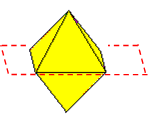#### You may also like### Reach for Polydron

A tetrahedron has two identical equilateral triangles faces, of side length 1 unit. The other two faces are right angled isosceles triangles. Find the exact volume of the tetrahedron.### Tetra Inequalities

Prove that in every tetrahedron there is a vertex such that the three edges meeting there have lengths which could be the sides of a triangle.### The Dodecahedron Explained

What is the shortest distance through the middle of a dodecahedron between the centres of two opposite faces?

# Octa-flower

##### Age 16 to 18Challenge LevelStart with a thought experiment in which you take some regular octahedra and, on each one, colour two faces that meet at a vertex but not along an edge. Now imagine taking two octahedra and gluing them together with coloured faces in contact so that the vertices where the coloured faces meet coincide. Now glue another octahedron on, coloured face to coloured face, so that the three vertices where the coloured faces meet coincide. Continue in the same way as long as possible. Calculate how many octahedra can be joined together in this way.